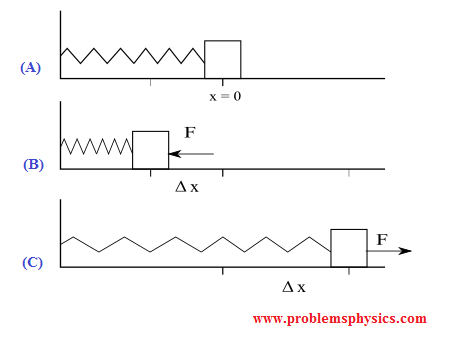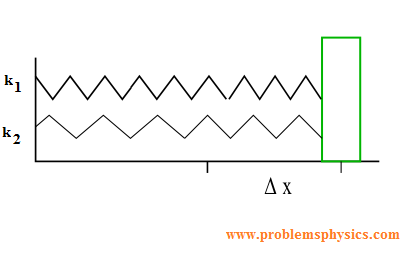# Hooke's Law, Examples with solutions

## Hooke's Law

In the diagram below is shown a block attached to a spring. In position (A) the spring is at rest and no external force acts on the block. In position (B) a force F is used to compress the spring by a length equal to Δ x by pushing the block to the left. In position (C), a force F is used to stretch the spring by a length Δ x by pulling the block to the right. Δ x is the change in the length of the spring measured from its position at rest.In both cases, the relationship between the magnitude of force F used to stretch or compress the spring by a length Δ x is given by Hooke's law as follows:
| F | = k | $\Delta x$ |

where k is the spring constant.
According to Newton's third law, if a spring is stretched or compressed using force F, as a reaction the spring also react by a force - F.

## Problems with Detailed Solutions

### Problem 1

What is the magnitude of the force required to stretch a 20 cm-long spring, with a spring constant of 100 N/m, to a length of 21 cm?
Solution
The spring changes from a length of 20 cm to 21 cm, hence it stretches by 1 cm or | $\Delta x$ | = 1 cm = 0.01 m.
| F | = k | $\Delta x$ | = 100 N / m × 0.01 m = 1 N

### Problem 2

What is the spring constant of a spring that needs a force of 3 N to be compressed from 40 cm to 35 cm?
Solution
The spring changes from a length of 40 cm to 35 cm, hence it stretches by 40 cm - 35 cm = 5 cm or | $\Delta x$ | = 5 cm = 0.05 m.
| F | = k | $\Delta x$ | = 3 N
k = | F | / |$\Delta x$| = 3 / 0.05 = 60 N / m

## Springs in Parallel

Two springs are said to be in parallel when used as in the figure below.The two springs behave like on spring whose constant k is given by
k = k1 + k2

### Problem 3

What is the magnitude of the force required to stretch two springs of constants k1 = 100 N / m and k2 = 200 N / m by 6 cm if they are in parallel?
Solution
The two springs behave like a spring with constant k given by
k = k1 + k2 = 100 N / m + 300 N / m
| F | = k | $\Delta x$ | = 300 N / m × 0.06 m = 18 N

## Spring in Series

Two springs are said to be in series when used as in the figure below.The two springs behave like on spring whose constant k is given by
1 / k = 1 / k1 + 1 / k2

### Problem 4

What is the magnitude of the force required to stretch two springs of constants 100 N / m and 200 N / m by 6 cm if they are in series?
Solution
The two springs behave like a spring with constant k obtained by solving for k the following
1 / k = 1 / 100 + 1 / 300
k = 75 N / m
| F | = k | $\Delta x$ | = 75 N / m × 0.06 m = 4.5 N

## Potential Energy of a Spring

To stretch or compress a spring by a length | $\Delta x$ |, energy is needed. Once stretched or compressed, the energy is stored in the spring as potential energy Pe and is given by:
Pe = (1/2) k $(\Delta x)^2$ , k is the spring constant. M

### Problem 5

How much energy W is needed to compress a spring from 15 cm to 10 cm if the constant of the spring is 150 N / m?
Solution
$\Delta x$ = 10 cm - 15 cm = - 5 cm = - 0.05 m
The energy W to compress the spring will all be stored as potential energy Pe in the spring, hence
W = Pe = (1/2) k $(\Delta x)^2$ = 0.5 × 150 × (- 0.05)2 = 0.1875 J